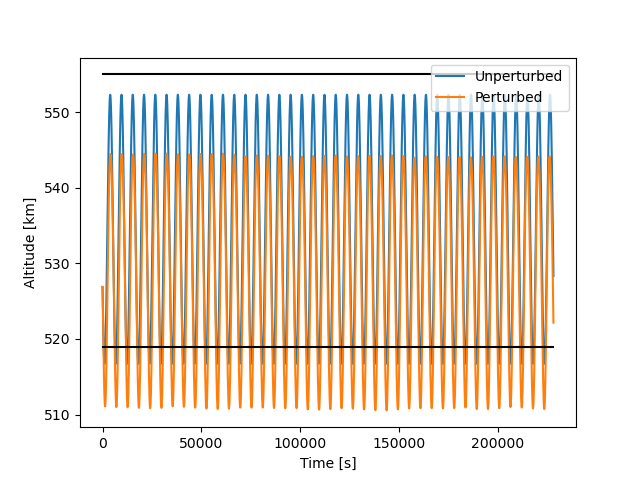# Inconsistent result from CDM

Hello everyone,

I noticed something weird. I’m trying to construct an orbit starting from position and velocity vectors reported in a CDM. However, I noticed that the orbit I obtain doesn’t match with the one reported in the CDM. For example, the perigee/apogee altitudes I obtain are like 15-20 km off.

The data in the CDM is the following:

``````EPHEMERIS_NAME                     =NONE
COVARIANCE_METHOD                  =CALCULATED
MANEUVERABLE                       =N/A
REF_FRAME                          =ITRF
GRAVITY_MODEL                      =EGM-96: 36D 36O
ATMOSPHERIC_MODEL                  =JBH09
N_BODY_PERTURBATIONS               =MOON,SUN
EARTH_TIDES                        =YES
INTRACK_THRUST                     =NO
COMMENT Covariance Scale Factor = 1.000000
COMMENT Exclusion Volume Radius = 5.000000 [m]
TIME_LASTOB_START                  =2022-06-14T17:25:22.284000
TIME_LASTOB_END                    =2022-06-15T17:25:22.284000
RECOMMENDED_OD_SPAN                =3.61                     [d]
ACTUAL_OD_SPAN                     =3.61                     [d]
OBS_AVAILABLE                      =77
OBS_USED                           =77
RESIDUALS_ACCEPTED                 =100                      [%]
WEIGHTED_RMS                       =1.046
COMMENT Apogee Altitude = 555   [km]
COMMENT Perigee Altitude = 519   [km]
COMMENT Inclination = 64.6  [deg]
AREA_PC                            =4.0115                   [m**2]
CD_AREA_OVER_MASS                  =0.058686041758           [m**2/kg]
CR_AREA_OVER_MASS                  =0.03235206892            [m**2/kg]
THRUST_ACCELERATION                =0                        [m/s**2]
SEDR                               =0.0035275                [W/kg]
X                                  =-4518.633435             [km]
Y                                  =-5197.753381             [km]
Z                                  =311.492934               [km]
X_DOT                              =1.898538049              [km/s]
Y_DOT                              =-2.038217158             [km/s]
Z_DOT                              =-6.861948694             [km/s]
COMMENT DCP Density Forecast Uncertainty = 2.558565910000000E-01
COMMENT DCP Sensitivity Vector RTN Pos = -1.254193830493978E+02 1.423044570895714E+04  -1.040300442687621E+01 [m]
COMMENT DCP Sensitivity Vector RTN Vel = -1.571039504748616E+01 2.408689885152749E-02  1.185898604331550E-03  [m/sec]
CR_R                               =1246.284324476203        [m**2]
CT_R                               =-121414.6354471415       [m**2]
CT_T                               =13852195.53971295        [m**2]
CN_R                               =12.69778964200831        [m**2]
CN_T                               =-9588.113401542814       [m**2]
CN_N                               =212.8639702159042        [m**2]
CRDOT_R                            =134.0091247376327        [m**2/s]
CRDOT_T                            =-15292.31649573428       [m**2/s]
CRDOT_N                            =10.60632150301289        [m**2/s]
CRDOT_RDOT                         =16.88245367896203        [m**2/s**2]
CTDOT_R                            =-0.4072702057806717      [m**2/s]
CTDOT_T                            =23.56698671492368        [m**2/s]
CTDOT_N                            =0.06416078696427642      [m**2/s]
CTDOT_RDOT                         =-0.02598128978827344     [m**2/s**2]
CTDOT_TDOT                         =0.0002636059025557587    [m**2/s**2]
CNDOT_R                            =-0.09248500369794832     [m**2/s]
CNDOT_T                            =1.080203506875406        [m**2/s]
CNDOT_N                            =-0.1932606908970702      [m**2/s]
CNDOT_RDOT                         =-0.001223627159008689    [m**2/s**2]
CNDOT_TDOT                         =0.00009394189797188796   [m**2/s**2]
CNDOT_NDOT                         =0.0004704721269538941    [m**2/s**2]
CDRG_R                             =0                        [m**3/kg]
CDRG_T                             =0                        [m**3/kg]
CDRG_N                             =0                        [m**3/kg]
CDRG_RDOT                          =0                        [m**3/(kg*s)]
CDRG_TDOT                          =0                        [m**3/(kg*s)]
CDRG_NDOT                          =0                        [m**3/(kg*s)]
CDRG_DRG                           =0                        [m**4/kg**2]
CSRP_R                             =0                        [m**3/kg]
CSRP_T                             =0                        [m**3/kg]
CSRP_N                             =0                        [m**3/kg]
CSRP_RDOT                          =0                        [m**3/(kg*s)]
CSRP_TDOT                          =0                        [m**3/(kg*s)]
CSRP_NDOT                          =0                        [m**3/(kg*s)]
CSRP_DRG                           =0                        [m**4/kg**2]
CSRP_SRP                           =0                        [m**4/kg**2]
``````

The first thing I do in my code is rotate the vectors into EME2000 as:

``````itrf2eme = FramesFactory.getITRF(IERSConventions.IERS_2010, True).getTransformTo(FramesFactory.getEME2000(), someDate);
r1v1 = itrf2eme.transformPVCoordinates(PVCoordinates(r1, v1));
``````

Then I use this rotated vectors as input to build the orbit as:

``````orbitSat = KeplerianOrbit(r1v1, FramesFactory.getEME2000(), someDate, Constants.WGS84_EARTH_MU);
``````

However, if I compute the perigee altitude as:

``````orbitSat.getA()*(1 - orbitSat.getE()) - Constants.WGS84_EARTH_EQUATORIAL_RADIUS
``````

The value I get is around 506 km while the CDM reports a value of 519 km. And even I use the Earth mean radius, the value doesn’t match either. The inclination is wrong too:

``````orbitSat.getI()*180/np.pi
``````

returns a value of 64.44 deg, while the CDM reports an inclination of 64.6 deg.
Does anyone have any suggestion?

Did you try using the full dynamical model described in the CDM,(i.e. gravity field, Sun and Moon effect, SRP) and look at the values over some time range.
Comparing just one osculating point and assuming Keplerian motion is perhaps not sufficient.

Hey there,

since these values are given as comments in the CDM, I would say that they could be in any pseudo-inertial reference frame. Have you tried TEME for example?

Edit: on second thought, they could even be from TLE data actually

Best,
Romain.

But the altitude of perigee/apogee shouldn’t depend on the reference frame (as long as the origin is always the same and as long as we’re talking about pseudo-inertial frames). Isn’t the only difference between TEME and EME the fact that the first accounts for nutation and precession of the spin axis?

I mean, that could explain the difference in the inclination, but not on the altitude. Indeed, the inclination in TEME is 64.55, which could match the 64.6 in the CDM (assuming the difference is only due to the rounding)

I did try that, the results are these:I propagated for 40 orbits.
The black lines represent the expected perigee and apogee altitudes.
As you can see the difference gets even worse.

Sidenote1: I’m now using the Earth mean radius (instead of the equatorial one) to calculate the altitude.
Sidenote2: since the CDM only provides `CD*area/mass`, `CR*area/mass`, and `area`, I assumed unitary mass, however I noticed that changing the value of the mass doesn’t affect the results that much.

TEME stands for True Equator Mean Equinox, so it is a strange mix of precession and nutation, the date to use is ambiguous, and its definition is not even official. Most people (including Orekit team) stand by David Vallado interpretation for this frame, though. Apart from this detail, you are right, the frame has the same origin as many other Earth based frames and distances should match.

As Luc explained, there is no universal definition of TEME. But yeah the impact should be on the inclination mostly.
These numbers in the comments definitely look rounded, that’s why they made me think of the SATCAT on space track. Hence my edit on the fact that they could well come from the last TLE (relative to the SGP4 propagator, maybe even in mean elements) of the object and thus not be completely consistent with the state vector within the CDM.
To be honest I would not worry too much about these relatively small differences you are seeing.

Romain

Yeah the object is indeed part of SATCAT in space-track. However I expected those comments to be consistent with the data in the CDMSo it’s somewhat “normal” to have differences between state vector and commented values?

Emiliano